JEE  >  Physics Test 9 - Electromagnetic Waves, Optics, Modern Physics

# Physics Test 9 - Electromagnetic Waves, Optics, Modern Physics

Test Description

## 25 Questions MCQ Test JEE Main & Advanced Mock Test Series | Physics Test 9 - Electromagnetic Waves, Optics, Modern Physics

Physics Test 9 - Electromagnetic Waves, Optics, Modern Physics for JEE 2023 is part of JEE Main & Advanced Mock Test Series preparation. The Physics Test 9 - Electromagnetic Waves, Optics, Modern Physics questions and answers have been prepared according to the JEE exam syllabus.The Physics Test 9 - Electromagnetic Waves, Optics, Modern Physics MCQs are made for JEE 2023 Exam. Find important definitions, questions, notes, meanings, examples, exercises, MCQs and online tests for Physics Test 9 - Electromagnetic Waves, Optics, Modern Physics below.
Solutions of Physics Test 9 - Electromagnetic Waves, Optics, Modern Physics questions in English are available as part of our JEE Main & Advanced Mock Test Series for JEE & Physics Test 9 - Electromagnetic Waves, Optics, Modern Physics solutions in Hindi for JEE Main & Advanced Mock Test Series course. Download more important topics, notes, lectures and mock test series for JEE Exam by signing up for free. Attempt Physics Test 9 - Electromagnetic Waves, Optics, Modern Physics | 25 questions in 60 minutes | Mock test for JEE preparation | Free important questions MCQ to study JEE Main & Advanced Mock Test Series for JEE Exam | Download free PDF with solutions
 1 Crore+ students have signed up on EduRev. Have you?
Physics Test 9 - Electromagnetic Waves, Optics, Modern Physics - Question 1

### A red LED emits light at 0.1 watt uniformly around it. The amplitude of the electric field of the light at a distance of 1 m from the diode is

Physics Test 9 - Electromagnetic Waves, Optics, Modern Physics - Question 2

### The resolution limit of the eye is 1 minute. At a distance of X km from the eye, two persons stand with a lateral separation of 3 meters. For the two persons to be just resolved by the naked eye, X should be

Physics Test 9 - Electromagnetic Waves, Optics, Modern Physics - Question 3

### In Young's double slit experiment, the fringe width is found to be 0.4 mm. If the whole apparatus is immersed in water of refractive index 4/3 without disturbing the geometrical arrangement, the new fringe width will be

Physics Test 9 - Electromagnetic Waves, Optics, Modern Physics - Question 4
For a prism of refractive index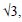the angle of the prism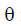is equal to the angle of minimum deviation.The value ofPhysics Test 9 - Electromagnetic Waves, Optics, Modern Physics - Question 5
Two optical media of refractive indices n1 and n2 contain x and y waves of the same colour in the same thickness. Then their relative refractive index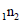is equal to
Physics Test 9 - Electromagnetic Waves, Optics, Modern Physics - Question 6
The focal length of a convex lens is 10 cm and its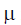is 1.5. If the radius of  curvature of one surface is 7.5 cm,the radius of curvature of the second surface will be
Physics Test 9 - Electromagnetic Waves, Optics, Modern Physics - Question 7
The refractive index of violet and red light are 1.54 and1.52 respectively. If the angle of prism is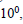the angular dispersion is
Physics Test 9 - Electromagnetic Waves, Optics, Modern Physics - Question 8
Interference fringes are obtained using two coherent sources whose intensities are in ratio 4 : 1. Then the ratio of the intensities of the bright and dark bands is
Physics Test 9 - Electromagnetic Waves, Optics, Modern Physics - Question 9
A ray of light passes through an equilateral prism such that the angle of incidence is equal to the angle of emergence and the latter equal to (3/4)th the angle of
the prism. The angle of deviation will be
Physics Test 9 - Electromagnetic Waves, Optics, Modern Physics - Question 10
A point object is placed at a distance of 10cm & its real image is formed at a distance of 20 cm from a concave mirror. If the object is moved by 0.1 cm towards the mirror, the image will shift by
Physics Test 9 - Electromagnetic Waves, Optics, Modern Physics - Question 11
Angle of a prism is A and its one surface is slivered.Light rays falling at an angle of incidence 2 A on first surface returns back, through, the same path after
suffering reflection at the second silvered surface.Refractive index of material is
Physics Test 9 - Electromagnetic Waves, Optics, Modern Physics - Question 12
Diamond shines due to its
Physics Test 9 - Electromagnetic Waves, Optics, Modern Physics - Question 13
When a beam of accelerated electrons hit a target a continuous X-ray spectrum is emitted from the target.Which one of these wavelengths is absent in the X-ray
spectrum if the X-rays tube is operated at 40,000V ?
Physics Test 9 - Electromagnetic Waves, Optics, Modern Physics - Question 14
The radio transmitter operates on a wavelength of 1500m at a power of 400 kilowatt. The energy of radiophoton in Joules is
Physics Test 9 - Electromagnetic Waves, Optics, Modern Physics - Question 15

What are the essential properties a medium must possess for the propagation of mechanical waves?

Detailed Solution for Physics Test 9 - Electromagnetic Waves, Optics, Modern Physics - Question 15

The friction force amongst the particles of the medium should be negligibly small so that they continue oscillating for a sufficiently long time and the wave travels a sufficiently long distance through the medium

Physics Test 9 - Electromagnetic Waves, Optics, Modern Physics - Question 16
The wavelength of the first line of Balmer series of hydrogen atom is l Å. The wavelength of this line of a double ionised lithium atom Z = 3) is
Physics Test 9 - Electromagnetic Waves, Optics, Modern Physics - Question 17
A freshly prepared radioactive source of half life 2 hrs emits radiations of intensity which is 64 times the permissible safe level. The minimum time after which it would be possible to work safely with this source is
Physics Test 9 - Electromagnetic Waves, Optics, Modern Physics - Question 18
A gamma ray photon creates an electron positron pair. If the rest mass energy of an electron is 0.5MeV and the total kinetic energy of the electron positron pair is 0.5 MeV, then the energy of the gamma ray photon must be
Physics Test 9 - Electromagnetic Waves, Optics, Modern Physics - Question 19
In the thermonuclear reaction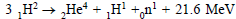the energy
released per nucleon of the reactants is
Physics Test 9 - Electromagnetic Waves, Optics, Modern Physics - Question 20

The relationship between α and β is given by

*Answer can only contain numeric values
Physics Test 9 - Electromagnetic Waves, Optics, Modern Physics - Question 21

A radiation energy 'E' falls normally on a perfectly reflecting surface. The momentum transferred to the surface is PE/C, then P is -

*Answer can only contain numeric values
Physics Test 9 - Electromagnetic Waves, Optics, Modern Physics - Question 22

Find the deviation of light ray (in degree)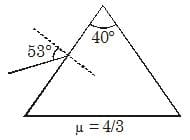Detailed Solution for Physics Test 9 - Electromagnetic Waves, Optics, Modern Physics - Question 22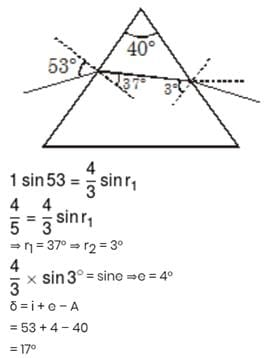*Answer can only contain numeric values
Physics Test 9 - Electromagnetic Waves, Optics, Modern Physics - Question 23

In the arrangement shown a point source of light S is placed 60 cm to the left of a convex lens of focal length 30 cm and 2 cm above the common principal axis of the convex lens and a convex mirror of focal length 10 cm. Consider optic centre of the lens to be the origin, the x-coordinate of the final image of the source S for the viewer left to the lens is equal to (– K) cm. Calculate the value of K.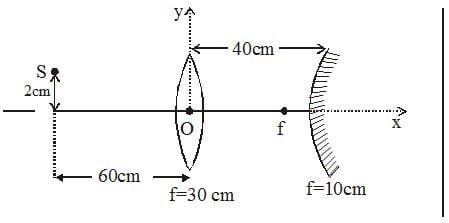Detailed Solution for Physics Test 9 - Electromagnetic Waves, Optics, Modern Physics - Question 23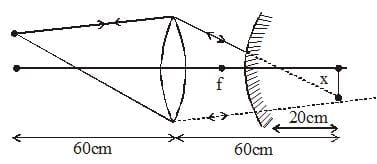*Answer can only contain numeric values
Physics Test 9 - Electromagnetic Waves, Optics, Modern Physics - Question 24

An isotropic point source of light is kept at distance of 60 cm from a screen, the intensity of light obtained on center is I1. Now a lens of focal length 20 cm is introduced at exactly midway between source and screen and intensity obtained is I2. Find I2/8I1.

Detailed Solution for Physics Test 9 - Electromagnetic Waves, Optics, Modern Physics - Question 24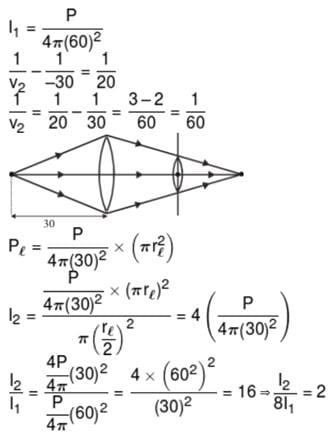*Answer can only contain numeric values
Physics Test 9 - Electromagnetic Waves, Optics, Modern Physics - Question 25

A wave of frequency 100 Hz travels along a string towards its fixed end. When this wave travels back, after reflection, a node is formed at a distance of 10 cm from the fixed end. The speed of the wave (incident and reflected) is :

Detailed Solution for Physics Test 9 - Electromagnetic Waves, Optics, Modern Physics - Question 25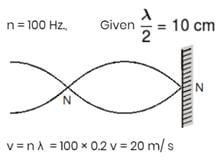## JEE Main & Advanced Mock Test Series

2 videos|325 docs|189 tests
 Use Code STAYHOME200 and get INR 200 additional OFF Use Coupon Code
Information about Physics Test 9 - Electromagnetic Waves, Optics, Modern Physics Page
In this test you can find the Exam questions for Physics Test 9 - Electromagnetic Waves, Optics, Modern Physics solved & explained in the simplest way possible. Besides giving Questions and answers for Physics Test 9 - Electromagnetic Waves, Optics, Modern Physics, EduRev gives you an ample number of Online tests for practice

## JEE Main & Advanced Mock Test Series

2 videos|325 docs|189 tests